# Resources tagged with: 2D shapes and their properties

Filter by: Content type:
Age range:
Challenge level:

There are 61 NRICH Mathematical resources connected to 2D shapes and their properties, you may find related items under Angles, Polygons, and Geometrical Proof.

Broad Topics > Angles, Polygons, and Geometrical Proof > 2D shapes and their properties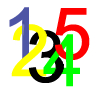### Track Design

##### Age 14 to 16Challenge Level

Where should runners start the 200m race so that they have all run the same distance by the finish?### Curvy Areas

##### Age 14 to 16Challenge Level

Have a go at creating these images based on circles. What do you notice about the areas of the different sections?### Trapezium Four

##### Age 14 to 16Challenge Level

The diagonals of a trapezium divide it into four parts. Can you create a trapezium where three of those parts are equal in area?### Spokes

##### Age 16 to 18Challenge Level

Draw three equal line segments in a unit circle to divide the circle into four parts of equal area.### Salinon

##### Age 14 to 16Challenge Level

This shape comprises four semi-circles. What is the relationship between the area of the shaded region and the area of the circle on AB as diameter?### Semi-detached

##### Age 14 to 16Challenge Level

A square of area 40 square cms is inscribed in a semicircle. Find the area of the square that could be inscribed in a circle of the same radius.### Approximating Pi

##### Age 14 to 18Challenge Level

By inscribing a circle in a square and then a square in a circle find an approximation to pi. By using a hexagon, can you improve on the approximation?##### Age 14 to 16Challenge Level

Given a square ABCD of sides 10 cm, and using the corners as centres, construct four quadrants with radius 10 cm each inside the square. The four arcs intersect at P, Q, R and S. Find the. . . .### Orthogonal Circle

##### Age 16 to 18Challenge Level

Given any three non intersecting circles in the plane find another circle or straight line which cuts all three circles orthogonally.### Logosquares

##### Age 16 to 18Challenge Level

Ten squares form regular rings either with adjacent or opposite vertices touching. Calculate the inner and outer radii of the rings that surround the squares.### Baby Circle

##### Age 16 to 18Challenge Level

A small circle fits between two touching circles so that all three circles touch each other and have a common tangent? What is the exact radius of the smallest circle?### Towering Trapeziums

##### Age 14 to 16Challenge Level

Can you find the areas of the trapezia in this sequence?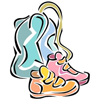### Gym Bag

##### Age 11 to 16Challenge Level

Can Jo make a gym bag for her trainers from the piece of fabric she has?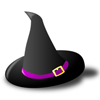### Witch's Hat

##### Age 11 to 16Challenge Level

What shapes should Elly cut out to make a witch's hat? How can she make a taller hat?### A Rational Search

##### Age 16 to 18Challenge Level

Investigate constructible images which contain rational areas.##### Age 14 to 16Challenge Level

Investigate the properties of quadrilaterals which can be drawn with a circle just touching each side and another circle just touching each vertex.### Efficient Packing

##### Age 14 to 16Challenge Level

How efficiently can you pack together disks?### Like a Circle in a Spiral

##### Age 7 to 16Challenge Level

A cheap and simple toy with lots of mathematics. Can you interpret the images that are produced? Can you predict the pattern that will be produced using different wheels?### Spirostars

##### Age 16 to 18Challenge Level

A spiropath is a sequence of connected line segments end to end taking different directions. The same spiropath is iterated. When does it cycle and when does it go on indefinitely?### LOGO Challenge 12 - Concentric Circles

##### Age 11 to 16Challenge Level

Can you reproduce the design comprising a series of concentric circles? Test your understanding of the realtionship betwwn the circumference and diameter of a circle.### LOGO Challenge 11 - More on Circles

##### Age 11 to 16Challenge Level

Thinking of circles as polygons with an infinite number of sides - but how does this help us with our understanding of the circumference of circle as pi x d? This challenge investigates. . . .### Gold Yet Again

##### Age 16 to 18Challenge Level

Nick Lord says "This problem encapsulates for me the best features of the NRICH collection."### First Forward Into Logo 4: Circles

##### Age 7 to 16Challenge Level

Learn how to draw circles using Logo. Wait a minute! Are they really circles? If not what are they?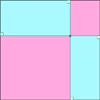### Pentagonal

##### Age 14 to 16Challenge Level

Can you prove that the sum of the distances of any point inside a square from its sides is always equal (half the perimeter)? Can you prove it to be true for a rectangle or a hexagon?### Lunar Angles

##### Age 16 to 18Challenge Level

What is the sum of the angles of a triangle whose sides are circular arcs on a flat surface? What if the triangle is on the surface of a sphere?### Circles in Circles

##### Age 16 to 18Challenge Level

This pattern of six circles contains three unit circles. Work out the radii of the other three circles and the relationship between them.##### Age 14 to 16Challenge Level

The sides of a triangle are 25, 39 and 40 units of length. Find the diameter of the circumscribed circle.### LOGO Challenge 8 - Rhombi

##### Age 7 to 16Challenge Level

Explore patterns based on a rhombus. How can you enlarge the pattern - or explode it?### LOGO Challenge 2 - Diamonds Are Forever

##### Age 7 to 16Challenge Level

The challenge is to produce elegant solutions. Elegance here implies simplicity. The focus is on rhombi, in particular those formed by jointing two equilateral triangles along an edge.### LOGO Challenge 6 - Triangles and Stars

##### Age 11 to 16Challenge Level

Recreating the designs in this challenge requires you to break a problem down into manageable chunks and use the relationships between triangles and hexagons. An exercise in detail and elegance.### LOGO Challenge - Circles as Animals

##### Age 11 to 16Challenge Level

See if you can anticipate successive 'generations' of the two animals shown here.### LOGO Challenge 10 - Circles

##### Age 11 to 16Challenge Level

In LOGO circles can be described in terms of polygons with an infinite (in this case large number) of sides - investigate this definition further.### Arclets Explained

##### Age 11 to 16

This article gives an wonderful insight into students working on the Arclets problem that first appeared in the Sept 2002 edition of the NRICH website.### Squaring the Circle and Circling the Square

##### Age 14 to 16Challenge Level

If you continue the pattern, can you predict what each of the following areas will be? Try to explain your prediction.### Semi-square

##### Age 14 to 16Challenge Level

What is the ratio of the area of a square inscribed in a semicircle to the area of the square inscribed in the entire circle?### Crescents and Triangles

##### Age 14 to 16Challenge Level

Can you find a relationship between the area of the crescents and the area of the triangle?### Pent

##### Age 14 to 18Challenge Level

The diagram shows a regular pentagon with sides of unit length. Find all the angles in the diagram. Prove that the quadrilateral shown in red is a rhombus.### Tricircle

##### Age 14 to 16Challenge Level

The centre of the larger circle is at the midpoint of one side of an equilateral triangle and the circle touches the other two sides of the triangle. A smaller circle touches the larger circle and. . . .### Rhombus in Rectangle

##### Age 14 to 16Challenge Level

Take any rectangle ABCD such that AB > BC. The point P is on AB and Q is on CD. Show that there is exactly one position of P and Q such that APCQ is a rhombus.### The Medieval Octagon

##### Age 14 to 16Challenge Level

Medieval stonemasons used a method to construct octagons using ruler and compasses... Is the octagon regular? Proof please.### Holly

##### Age 14 to 16Challenge Level

The ten arcs forming the edges of the "holly leaf" are all arcs of circles of radius 1 cm. Find the length of the perimeter of the holly leaf and the area of its surface.### Three Four Five

##### Age 14 to 16Challenge Level

Two semi-circles (each of radius 1/2) touch each other, and a semi-circle of radius 1 touches both of them. Find the radius of the circle which touches all three semi-circles.### Tied Up

##### Age 14 to 16 ShortChallenge Level

How much of the field can the animals graze?### Floored

##### Age 14 to 16Challenge Level

A floor is covered by a tessellation of equilateral triangles, each having three equal arcs inside it. What proportion of the area of the tessellation is shaded?### Circle Packing

##### Age 14 to 16Challenge Level

Equal circles can be arranged so that each circle touches four or six others. What percentage of the plane is covered by circles in each packing pattern? ...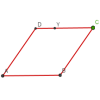### Roaming Rhombus

##### Age 14 to 16Challenge Level

We have four rods of equal lengths hinged at their endpoints to form a rhombus ABCD. Keeping AB fixed we allow CD to take all possible positions in the plane. What is the locus (or path) of the point. . . .### From All Corners

##### Age 14 to 16Challenge Level

Straight lines are drawn from each corner of a square to the mid points of the opposite sides. Express the area of the octagon that is formed at the centre as a fraction of the area of the square.### Dividing the Field

##### Age 14 to 16Challenge Level

A farmer has a field which is the shape of a trapezium as illustrated below. To increase his profits he wishes to grow two different crops. To do this he would like to divide the field into two. . . .### Escriptions

##### Age 16 to 18Challenge Level

For any right-angled triangle find the radii of the three escribed circles touching the sides of the triangle externally.### Retracircles

##### Age 16 to 18Challenge Level

Four circles all touch each other and a circumscribing circle. Find the ratios of the radii and prove that joining 3 centres gives a 3-4-5 triangle.